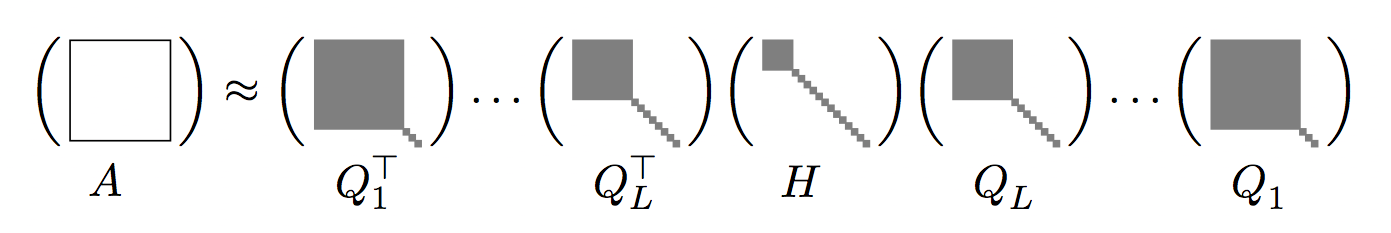# Multiresolution matrix factorizationsMost commonly used matrix factorization algorithms, such as eigendecomposition (PCA), singular value decomposition (SVD), or non-negative matrix factorization (NMF) are inherently single-level algorithms. For example, PCA decomposes a symmetric matrix $$A$$ in the form $A=Q^\top\! D\,Q,$ where $$Q$$ is an orthogonal matrix and $$D$$ is diagonal. In general, the rows of $$Q$$ are dense. When $$A$$ is a massive matrix coming from real world data, this becomes problematic, both from the computational point of view and from the point of view of interpretability.

Our paper [P1] reformulated matrix factorization as a type of multiresolution analysis on a metric space, and used this connection to define a generic notion of Multiresolution Matrix Factorization (MMF) of the form $A= Q_1^\top Q_2^\top\ldots Q_L^\top H Q_L\ldots Q_2 Q_1,$ where the $$Q_1,Q_2,\ldots,Q_L$$ orthogonal matrices are very sparse and $$H$$ is close to diagonal. Subsequently, in [P2] we generalized the algorithm to a parallel setting and showed how it can produce state-of-the-art results on matrix compression tasks in close to linear time in the number of non-zeros [P3]. Currently, we are working on applying the MMF formalism to speed up large scale numerical computations including Gaussian process inference [P5] and solving linear systems [p6].

MMF is related to well established numerical analysis paradigms, such as hierarchical matrices and the fast multipole method. Potentially, it can be used to accelerate a wide range of large linear algebra tasks. However, this required writing a dedicated, high-performance, multicore implementation, which we called pMMF, from scratch [S1]. The project necessitated writing our own blocked sparse matrix library, which subsequently took on a life of its own under the name Mondrian [S2].

### Papers

[P1]  R. Kondor, N. Teneva and V. Garg:  Multiresolution matrix factorization (ICML 2014)  [paper] [supplement] [video]

[P2]  R. Kondor, N. Teneva and P. K. Mudrakarta:  Parallel MMF: a multiresolution approach to matrix computation (arXiv, July 2015)  [preprint]

[P3]  N. Teneva, P. K. Mudrakarta and R. Kondor:  Multiresolution Matrix Compression (AISTATS 2016) (winner of notable student paper award)  [paper]

[P4]  V. Ithapu, R. Kondor and V. Singh:  The Incremental Multiresolution Matrix Factorization Algorithm (CVPR 2017)

[P5]  Y. Ding, R. Kondor and J. Eskreis-Winkler:  Multiresolution kernel approximation for Gaussian process regression (NIPS 2017)  [preprint]

### Software

[S1]  R. Kondor, N. Teneva and P. K. Mudrakarta:  pMMF: a high performance C++ library for parallel multiresolution matrix factorization  [pMMF]

[S2]  R. Kondor:  Mondrian:  C++ parallel blocked matrix library  [Mondrian]

### Events

I. Dhillon, R. Kondor, R. Nowak, M. O'Neil and N. Teneva: Multiresolution Methods for Large Scale Learning (NIPS 2015 workshop)  [schedule] NIPS 2015 [videos]